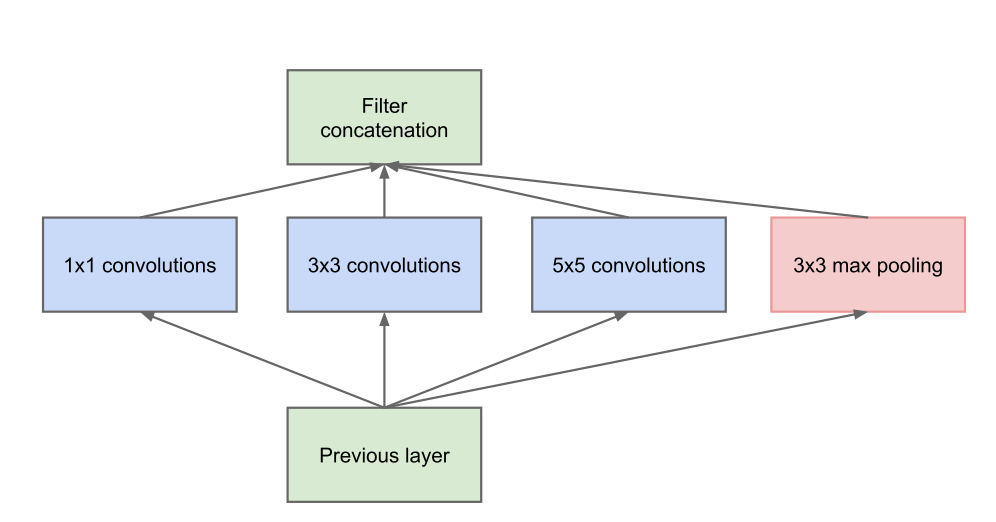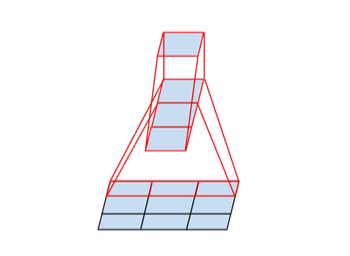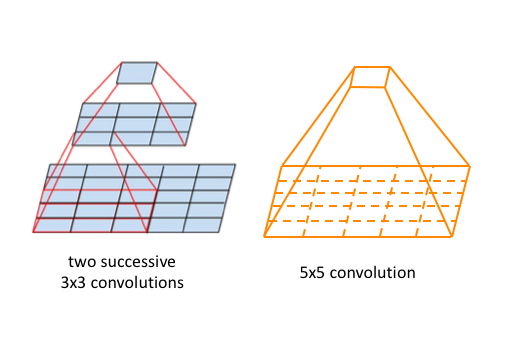# v1.5.3

Version 1.5.3 of DynaML, released August 14, 2017, introduces a new API for handling data sets. It also features greater TensorFlow related integrations, notably the Inception v2 cell.

### Data Set API¶

The DataSet family of classes helps the user to create and transform potentially large number of data instances. Users can create and perform complex transformations on data sets, using the DataPipe API or simple Scala functions.

import _root_.io.github.mandar2812.dynaml.probability._
import _root_.io.github.mandar2812.dynaml.pipes._
import io.github.mandar2812.dynaml.tensorflow._

val random_numbers = GaussianRV(0.0, 1.0) :* GaussianRV(1.0, 2.0)

//Create a data set.
val dataset1 = dtfdata.dataset(random_numbers.iid(10000).draw)

val filter_gr_zero = DataPipe[(Double, Double), Boolean](
c => c._1 > 0d && c._2 > 0d
)

//Filter elements
val data_gr_zero = dataset1.filter(filter_gr_zero)

val abs_func: (Double, Double) => (Double, Double) =
(c: (Double, Double)) => (math.abs(c._1), math.abs(c._2))

//Map elements
val data_abs     = dataset1.map(abs_func)


Find out more about the DataSet API and its capabilities in the user guide.

### Tensorflow Integration¶

Package dynaml.tensorflow

#### Batch Normalisation¶

Batch normalisation is used to standardize activations of convolutional layers and to speed up training of deep neural nets.

Usage

import io.github.mandar2812.dynaml.tensorflow._

val bn = dtflearn.batch_norm("BatchNorm1")


#### Inception v2¶

The Inception architecture, proposed by Google is an important building block of convolutional neural network architectures used in vision applications.In a subsequent paper, the authors introduced optimizations in the Inception architecture, known colloquially as Inception v2.

In Inception v2, larger convolutions (i.e. 3 x 3 and 5 x 5) are implemented in a factorized manner to reduce the number of parameters to be learned. For example the 3 x 3 convolution is expressed as a combination of 1 x 3 and 3 x 1 convolutions.Similarly the 5 x 5 convolutions can be expressed a combination of two 3 x 3 convolutionsDynaML now offers the Inception cell as a computational layer.

Usage

import io.github.mandar2812.dynaml.pipes._
import io.github.mandar2812.dynaml.tensorflow._
import org.platanios.tensorflow.api._

//Create an RELU activation, given a string name/identifier.
val relu_act = DataPipe(tf.learn.ReLU(_))

//Learn 10 filters in each branch of the inception cell
val filters = Seq(10, 10, 10, 10)

val inception_cell = dtflearn.inception_unit(
channels = 3,  num_filters = filters, relu_act,
//Apply batch normalisation after each convolution
use_batch_norm = true)(layer_index = 1)


#### Dynamical Systems: Continuous Time RNN¶

Continuous time recurrent neural networks (CTRNN) are an important class of recurrent neural networks. They enable the modelling of non-linear and potentially complex dynamical systems of multiple variables, with feedback.

• Added CTRNN layer: dtflearn.ctrnn

• Added CTRNN layer with inferable time step: dtflearn.dctrnn.

• Added a projection layer for CTRNN based models dtflearn.ts_linear.

Training Stopping Criteria

Create common and simple training stop criteria such as.

• Stop after fixed number of iterations dtflearn.max_iter_stop(100000)

• Stop after change in value of loss goes below a threshold. dtflearn.abs_loss_change_stop(0.0001)

• Stop after change in relative value of loss goes below a threshold. dtflearn.rel_loss_change_stop(0.001)

Neural Network Building Blocks

• Added helper method dtlearn.build_tf_model() for training tensorflow models/estimators.

Usage

import io.github.mandar2812.dynaml.tensorflow._
import org.platanios.tensorflow.api._
import ammonite.ops._

val tempdir = home/"tmp"

java.nio.file.Paths.get(tempdir.toString())
)

val trainImages = tf.data.TensorSlicesDataset(dataSet.trainImages)
val trainLabels = tf.data.TensorSlicesDataset(dataSet.trainLabels)

val trainData =
trainImages.zip(trainLabels)
.repeat()
.shuffle(10000)
.batch(256)
.prefetch(10)

// Create the MLP model.
val input = tf.learn.Input(
UINT8,
Shape(
-1,
dataSet.trainImages.shape(1),
dataSet.trainImages.shape(2))
)

val trainInput = tf.learn.Input(UINT8, Shape(-1))

val architecture = tf.learn.Flatten("Input/Flatten") >>
tf.learn.Cast("Input/Cast", FLOAT32) >>
tf.learn.Linear("Layer_0/Linear", 128) >>
tf.learn.ReLU("Layer_0/ReLU", 0.1f) >>
tf.learn.Linear("Layer_1/Linear", 64) >>
tf.learn.ReLU("Layer_1/ReLU", 0.1f) >>
tf.learn.Linear("Layer_2/Linear", 32) >>
tf.learn.ReLU("Layer_2/ReLU", 0.1f) >>
tf.learn.Linear("OutputLayer/Linear", 10)

val trainingInputLayer = tf.learn.Cast("TrainInput/Cast", INT64)

val loss =
tf.learn.SparseSoftmaxCrossEntropy("Loss/CrossEntropy") >>
tf.learn.Mean("Loss/Mean") >>
tf.learn.ScalarSummary("Loss/Summary", "Loss")

// Directory in which to save summaries and checkpoints
val summariesDir = java.nio.file.Paths.get(
(tempdir/"mnist_summaries").toString()
)

val (model, estimator) = dtflearn.build_tf_model(
architecture, input, trainInput, trainingInputLayer,
loss, optimizer, summariesDir, dtflearn.max_iter_stop(1000),
100, 100, 100)(trainData)

• Build feedforward layers and feedforward layer stacks easier.

Usage

import io.github.mandar2812.dynaml.tensorflow._
import org.platanios.tensorflow.api._

//Create a single feedforward layer
val layer = dtflearn.feedforward(num_units = 10, useBias = true)(id = 1)

//Create a stack of feedforward layers

val net_layer_sizes = Seq(10, 5, 3)

val stack = dtflearn.feedforward_stack(
(i: Int) => dtflearn.Phi("Act_"+i), FLOAT64)(
net_layer_sizes)


### 3D Graphics¶

Package dynaml.graphics

Create 3d plots of surfaces, for a use case, see the jzydemo.sc and tf_wave_pde.sc

### Library Organisation¶

• Removed the dynaml-notebook module.

## Bug Fixes¶

• Fixed bug related to scalar method of VectorField, innerProdDouble and other inner product implementations.

• RegressionMetrics and RegressionMetricsTF now also compute Spearman rank correlation as one of the performance metrics.